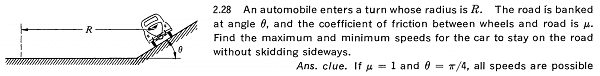# Car turning without skidding

## Homework StatementF = ma

## The Attempt at a Solution

First off, to keep the car from skidding to the side we want it to be constrained to move in a circle about the center of the turn because if it skids then it has deviated / veered off tangentially at some point along a circular path. For the minimum velocity, we want friction to point in the direction away from the center so that the net centripetal force is less thus requiring a minimal velocity. The equations of motion for the car are $Nsin\theta - fcos\theta = M\frac{v_{min}^{2}}{R}, Ncos\theta + fsin\theta - Mg = 0$ which tells us that $v_{min}^{2} = gR\frac{tan\theta - \mu }{1 + \mu tan\theta }$. For the maximum case we just negate the direction of friction and this gives us, in a very similar way, $v_{max}^{2} = gR\frac{tan\theta + \mu }{1 - \mu tan\theta }$. Plugging in the parameters give in the ans. clue we see that $v_{min} = 0, v_{max} = \infty$ so any speed in the range $0 \leq v< \infty$ will keep it from skidding which is all possible speeds. Is that what the text means by "all speeds are possible"? Thanks!

haruspex
Science Advisor
Homework Helper
Gold Member
2020 Award
Yes.